International
Tables for
Crystallography
Volume B
Reciprocal space
Edited by U. Shmueli

International Tables for Crystallography (2006). Vol. B, ch. 5.2, pp. 555-556   | 1 | 2 |

## Section 5.2.13. Born series

A. F. Moodie,a J. M. Cowleyb and P. Goodmanc

aDepartment of Applied Physics, Royal Melbourne Institute of Technology, 124 La Trobe Street, Melbourne, Victoria 3000, Australia,bArizona State University, Box 871504, Department of Physics and Astronomy, Tempe, AZ 85287-1504, USA, and cSchool of Physics, University of Melbourne, Parkville, Australia 3052

### 5.2.13. Born series

| top | pdf |

In the impulse limit of equation (5.2.12.2), the integrals can be evaluated to give the Born series (Cowley & Moodie, 1957)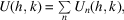where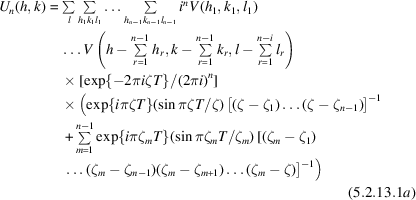and where n is the order of interaction. Hereis the excitation error of the reflection with index h, k, and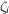are the excitation errors for the reflections with indices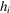,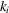,. Thus each constituent process may be represented by a diagram, starting on the origin of reciprocal space, possibly looped, and ending on the point with coordinates (hk).

This solution can also be obtained by iteration of the Green-function integral equation, the integrals being evaluated by means of suitably chosen contours on the complexplane (Fujiwara, 1959), as well as by expansion of the scattering matrix (Fujimoto, 1959).

Clearly, two or more of thewill, in general, be equal in nearly all of the terms in equation (5.2.13.1a). Confluence is, however, readily described, the divided differences of arbitrary order transforming into differentials of the same order (Moodie, 1972).

The physical picture that emerges from equation (5.2.13.1a) is that of n-fold scattering, the initial wave being turned through n − 1 intermediate states, processes that can be presented by scattering diagrams in reciprocal space (Gjønnes & Moodie, 1965).

For a given scattering vector, constituent functions are evaluated for all possible paths in three dimensions, and those functions are then summed over l. There are therefore two distinct processes by which upper-layer lines can perturb wavefunctions in the zone, namely: by scattering out of the zone and then back in; and by intrusion of the effective shape function from another zone, the latter process being already operative in the first Born, or kinematical approximation.

The constituent functions to be evaluated can be transformed into many forms. One of the more readily described is that which assigns to each diagram an effective dynamical shape function. If there are no loops in the diagram of order n, this effective shape function is the (n + 1)th divided difference of the constituent phase-shifted kinematical shape transforms. For general diagrams, divided differences in loops are replaced by the corresponding differentials. The resulting function is multiplied by the convolution of the contributing structure amplitudes and diagrams of all orders summed (Moodie, 1972).

While scattering diagrams have no utility in numerical work, they find application in the analysis of symmetries, for instance in the determination of the presence or absence of a centre of inversion [for a recent treatment, see Moodie & Whitfield (1995)] and in the detection of screw axes and glide planes (Gjønnes & Moodie, 1965). Methods for the direct determination of all space groups are described by Goodman (1975) and by Tanaka et al. (1983) (see Section 2.5.3).

Equation (5.2.13.1a) can be rewritten in a form particularly suited to the classification of approximations, and to describing the underlying symmetry of the formulation. The equation is written for compactness as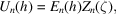so that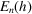depends only on crystal structure and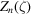only on diffraction geometry. A transformation (Cowley & Moodie, 1962) involving bialternants leads to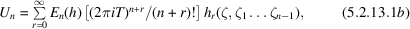where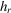is the complete homogeneous symmetric polynomial function of n variables of order r.

Upper-layer-line effects can, of course, be calculated in any of the formulations.

### References

Cowley, J. M. & Moodie, A. F. (1957). The scattering of electrons by atoms and crystals. I. A new theoretical approach. Acta Cryst. 10, 609–619.Google Scholar
Cowley, J. M. & Moodie, A. F. (1962). The scattering of electrons by thin crystals. J. Phys. Soc. Jpn, 17, Suppl. B11, 86–91.Google Scholar
Fujimoto, F. (1959). Dynamical theory of electron diffraction in the Laue case. J. Phys. Soc. Jpn, 14, 1558–1568.Google Scholar
Fujiwara, K. (1959). Application of higher order Born approximation to multiple elastic scattering of electrons by crystals. J. Phys. Soc. Jpn, 14, 1513–1524.Google Scholar
Gjønnes, J. & Moodie, A. F. (1965). Extinction conditions in the dynamic theory of electron diffraction. Acta Cryst. 19, 65–67.Google Scholar
Goodman, P. (1975). A practical method of three-dimensional space-group analysis using convergent-beam electron diffraction. Acta Cryst. A31, 804–810.Google Scholar
Moodie, A. F. (1972). Reciprocity and shape functions in multiple scattering diagrams. Z. Naturforsch. Teil A, 27, 437–440.Google Scholar
Moodie, A. F. & Whitfield, H. J. (1995). Friedel's law and non-centrosymmetric space groups. Acta Cryst. A51, 198–201.Google Scholar
Tanaka, M., Sekii, H. & Nagasawa, T. (1983). Space-group determination by dynamic extinction in convergent-beam electron diffraction. Acta Cryst. A39, 825–837.Google Scholar